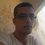# Finding a counter-example for Polya's conjecture.

Before we start, let us define two functions, let $$n$$ a positive integer and $$m$$ is the number of total prime divisors of $$n$$ (for example, if $$n=12$$ then $$m=|\{2,2,3\}|=3$$, and we define $$\lambda(n)=(-1)^m$$. And also : $L(n)=\sum_{k=1}^{n} \lambda(k)$ Polya conjectured that $$L(n)\leq 0$$ for any $$n\geq 2$$, but this was disproved fifty years ago and the counter example was a very large number (almost a billion).

Our task : let us assume that we do not know that there is a counter-example and we try to find one, what do you suggest as the best programming language for this ? and how can we speed up the search for a counter example ?

Please share your codes and running time and the machine specifications and remember that they made it work on a 1958 computer, it should be doable on a 2014 computer.

Wiki page :Polya's conjecture.Note by Haroun Meghaichi
6 years, 8 months ago

This discussion board is a place to discuss our Daily Challenges and the math and science related to those challenges. Explanations are more than just a solution — they should explain the steps and thinking strategies that you used to obtain the solution. Comments should further the discussion of math and science.

When posting on Brilliant:

• Use the emojis to react to an explanation, whether you're congratulating a job well done , or just really confused .
• Ask specific questions about the challenge or the steps in somebody's explanation. Well-posed questions can add a lot to the discussion, but posting "I don't understand!" doesn't help anyone.
• Try to contribute something new to the discussion, whether it is an extension, generalization or other idea related to the challenge.
• Stay on topic — we're all here to learn more about math and science, not to hear about your favorite get-rich-quick scheme or current world events.

MarkdownAppears as
*italics* or _italics_ italics
**bold** or __bold__ bold
- bulleted- list
• bulleted
• list
1. numbered2. list
1. numbered
2. list
Note: you must add a full line of space before and after lists for them to show up correctly
paragraph 1paragraph 2

paragraph 1

paragraph 2

[example link](https://brilliant.org)example link
> This is a quote
This is a quote
    # I indented these lines
# 4 spaces, and now they show
# up as a code block.

print "hello world"
# I indented these lines
# 4 spaces, and now they show
# up as a code block.

print "hello world"
MathAppears as
Remember to wrap math in $$ ... $$ or $ ... $ to ensure proper formatting.
2 \times 3 $2 \times 3$
2^{34} $2^{34}$
a_{i-1} $a_{i-1}$
\frac{2}{3} $\frac{2}{3}$
\sqrt{2} $\sqrt{2}$
\sum_{i=1}^3 $\sum_{i=1}^3$
\sin \theta $\sin \theta$
\boxed{123} $\boxed{123}$

## Comments

Sort by:

Top Newest

Vous n'êtes pas mauvais en maths, êtes-vous? Je voudrais avoir le temps de travailler sur ce plus mais je n'ai pas. Il est très intéressant!

- 6 years, 8 months ago

Log in to reply

I didn't know that you speak French! Ce problème est très intéressant car les solutions classique utilise beaucoup de mémoire RAM (par exemple l'utilisation des cribles). Tant que les RAMs des années cinquante étaient au plus 500 mega, je crois qu'il y a des optimisation majeures qui peut être mise ici.

Anyway, thanks for replying. And for your question : I'm not good too.

- 6 years, 8 months ago

Log in to reply

×

Problem Loading...

Note Loading...

Set Loading...